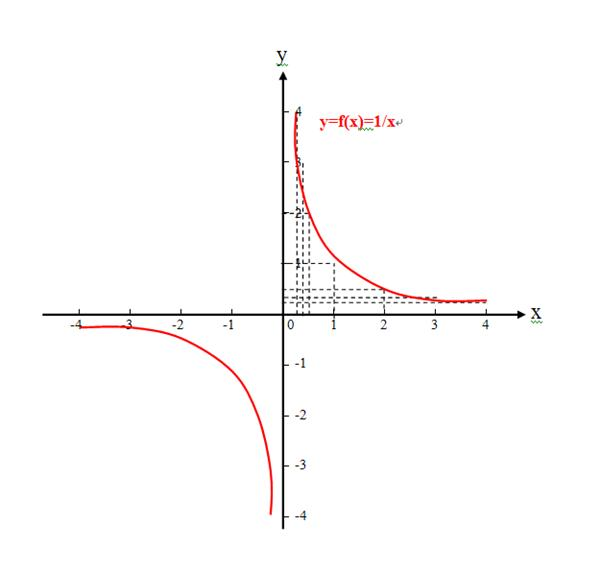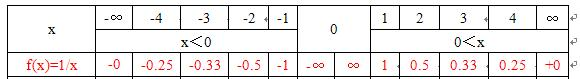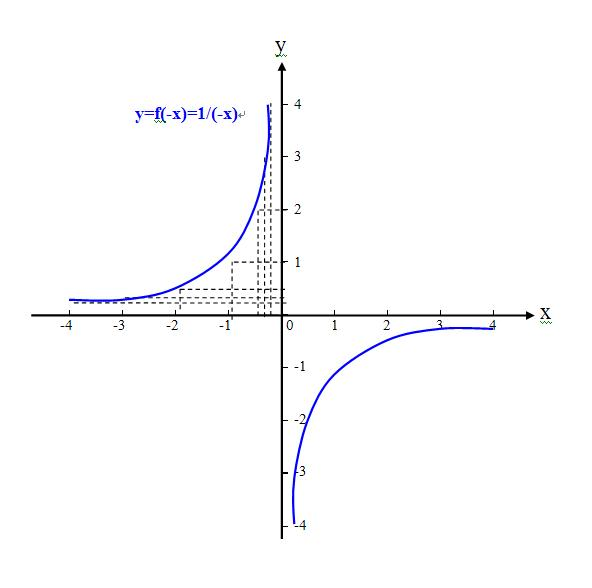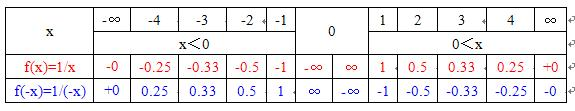### 寒湘子举例函数f(x)=1/x和函数f(-x)=1/(-x)，是不是一个函数？

 标签(TAG)： 下来我讨论一下，寒湘子举例函数f(x)=1/x和函数f(-x)=1/(-x)，是不是一个函数？ 1、已知函数f(x)=1/x，如图2、依据已知函数f(x)=1/x，求得函数f(-x)=1/(-x)，如图3、寒湘子说“函数的三个要素是定义域、值域、对应关系”，那么函数f(x)=1/x和函数f(-x)=1/(-x)是不是一个函数？ 1）函数f(x)=1/x的对应关系是f( )=1/( )，函数f(-x)=1/(-x) 的对应关系是f(-( ) )=1/(-( ) )，所以要素之一“对应关系”不同，后者对自变量x有一个求反的过程； 2）显然，函数f(x)=1/x和函数f(-x)=1/(-x)不是一个函数，而是两个函数； 3）不过，函数f(x)=1/x和函数f(-x)=1/(-x)是两个相关函数，函数f(x)=1/x是已知函数，函数f(-x)=1/(-x)是由已知函数f(x)=1/x对自变量x求反得出的，图像是与已知函数图像以y轴为对称的； 4）不知寒湘子举此例，并没有说明白函数f(x)=1/x和函数f(-x)=1/(-x)是一个函数，想说明什么问题呢？ 4、这个问题搞清楚了，主楼的题目就会迎刃而解，就知道f(-x)并不是已知函数f(x)图像y轴左侧的一个函数值，就不会再想着设法给已知函数补充另一半定义区间及其函数，……，就不会再出笑话！ .